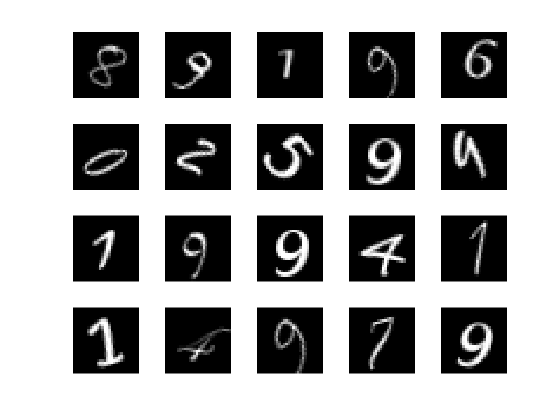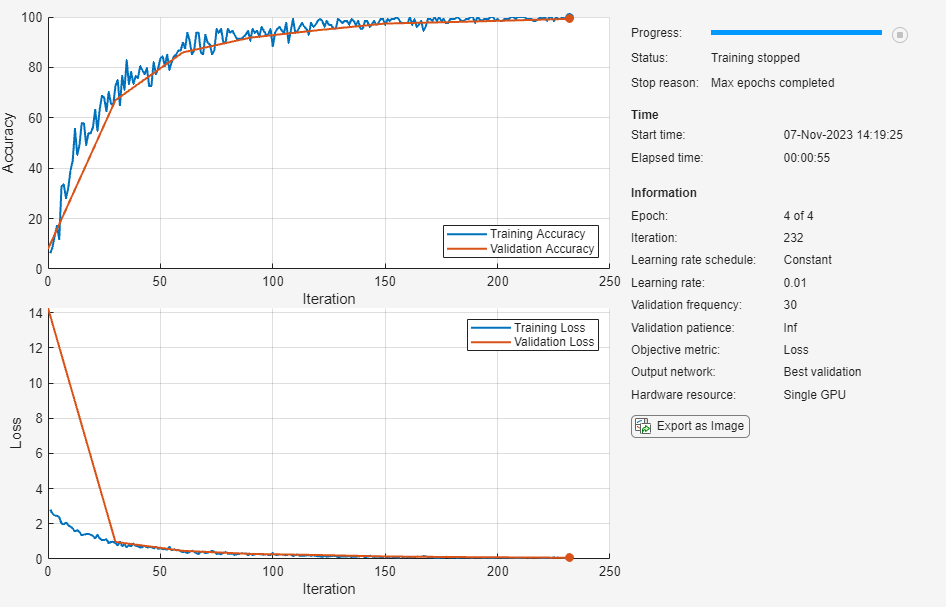# Create Simple Deep Learning Neural Network for Classification

This example shows how to create and train a simple convolutional neural network for deep learning classification. Convolutional neural networks are essential tools for deep learning, and are especially suited for image recognition.

The example demonstrates how to:

• Load and explore image data.

• Define the neural network architecture.

• Specify training options.

• Train the neural network.

• Predict the labels of new data and calculate the classification accuracy.

For an example showing how to interactively create and train a simple image classification neural network, see Create Simple Image Classification Network Using Deep Network Designer.

### Load and Explore Image Data

Load the digit sample data as an image datastore. `imageDatastore` automatically labels the images based on folder names and stores the data as an `ImageDatastore` object. An image datastore enables you to store large image data, including data that does not fit in memory, and efficiently read batches of images during training of a convolutional neural network.

```digitDatasetPath = fullfile(matlabroot,'toolbox','nnet','nndemos', ... 'nndatasets','DigitDataset'); imds = imageDatastore(digitDatasetPath, ... 'IncludeSubfolders',true,'LabelSource','foldernames');```

Display some of the images in the datastore.

```figure; perm = randperm(10000,20); for i = 1:20 subplot(4,5,i); imshow(imds.Files{perm(i)}); end```Calculate the number of images in each category. `labelCount` is a table that contains the labels and the number of images having each label. The datastore contains 1000 images for each of the digits 0-9, for a total of 10000 images. You can specify the number of classes in the last fully connected layer of your neural network as the `OutputSize` argument.

`labelCount = countEachLabel(imds)`
```labelCount=10×2 table Label Count _____ _____ 0 1000 1 1000 2 1000 3 1000 4 1000 5 1000 6 1000 7 1000 8 1000 9 1000 ```

You must specify the size of the images in the input layer of the neural network. Check the size of the first image in `digitData`. Each image is 28-by-28-by-1 pixels.

```img = readimage(imds,1); size(img)```
```ans = 1×2 28 28 ```

### Specify Training and Validation Sets

Divide the data into training and validation data sets, so that each category in the training set contains 750 images, and the validation set contains the remaining images from each label. `splitEachLabel` splits the datastore `digitData` into two new datastores, `trainDigitData` and `valDigitData`.

```numTrainFiles = 750; [imdsTrain,imdsValidation] = splitEachLabel(imds,numTrainFiles,'randomize');```

### Define Neural Network Architecture

Define the convolutional neural network architecture.

```layers = [ imageInputLayer([28 28 1]) convolution2dLayer(3,8,'Padding','same') batchNormalizationLayer reluLayer maxPooling2dLayer(2,'Stride',2) convolution2dLayer(3,16,'Padding','same') batchNormalizationLayer reluLayer maxPooling2dLayer(2,'Stride',2) convolution2dLayer(3,32,'Padding','same') batchNormalizationLayer reluLayer fullyConnectedLayer(10) softmaxLayer classificationLayer];```

Image Input Layer An `imageInputLayer` is where you specify the image size, which, in this case, is 28-by-28-by-1. These numbers correspond to the height, width, and the channel size. The digit data consists of grayscale images, so the channel size (color channel) is 1. For a color image, the channel size is 3, corresponding to the RGB values. You do not need to shuffle the data because `trainNetwork`, by default, shuffles the data at the beginning of training. `trainNetwork` can also automatically shuffle the data at the beginning of every epoch during training.

Convolutional Layer In the convolutional layer, the first argument is `filterSize`, which is the height and width of the filters the training function uses while scanning along the images. In this example, the number 3 indicates that the filter size is 3-by-3. You can specify different sizes for the height and width of the filter. The second argument is the number of filters, `numFilters`, which is the number of neurons that connect to the same region of the input. This parameter determines the number of feature maps. Use the `'Padding'` name-value pair to add padding to the input feature map. For a convolutional layer with a default stride of 1, `'same'` padding ensures that the spatial output size is the same as the input size. You can also define the stride and learning rates for this layer using name-value pair arguments of `convolution2dLayer`.

Batch Normalization Layer Batch normalization layers normalize the activations and gradients propagating through a neural network, making neural network training an easier optimization problem. Use batch normalization layers between convolutional layers and nonlinearities, such as ReLU layers, to speed up neural network training and reduce the sensitivity to neural network initialization. Use `batchNormalizationLayer` to create a batch normalization layer.

ReLU Layer The batch normalization layer is followed by a nonlinear activation function. The most common activation function is the rectified linear unit (ReLU). Use `reluLayer` to create a ReLU layer.

Max Pooling Layer Convolutional layers (with activation functions) are sometimes followed by a down-sampling operation that reduces the spatial size of the feature map and removes redundant spatial information. Down-sampling makes it possible to increase the number of filters in deeper convolutional layers without increasing the required amount of computation per layer. One way of down-sampling is using a max pooling, which you create using `maxPooling2dLayer`. The max pooling layer returns the maximum values of rectangular regions of inputs, specified by the first argument, `poolSize`. In this example, the size of the rectangular region is [2,2]. The `'Stride'` name-value pair argument specifies the step size that the training function takes as it scans along the input.

Fully Connected Layer The convolutional and down-sampling layers are followed by one or more fully connected layers. As its name suggests, a fully connected layer is a layer in which the neurons connect to all the neurons in the preceding layer. This layer combines all the features learned by the previous layers across the image to identify the larger patterns. The last fully connected layer combines the features to classify the images. Therefore, the `OutputSize` parameter in the last fully connected layer is equal to the number of classes in the target data. In this example, the output size is 10, corresponding to the 10 classes. Use `fullyConnectedLayer` to create a fully connected layer.

Softmax Layer The softmax activation function normalizes the output of the fully connected layer. The output of the softmax layer consists of positive numbers that sum to one, which can then be used as classification probabilities by the classification layer. Create a softmax layer using the `softmaxLayer` function after the last fully connected layer.

Classification Layer The final layer is the classification layer. This layer uses the probabilities returned by the softmax activation function for each input to assign the input to one of the mutually exclusive classes and compute the loss. To create a classification layer, use `classificationLayer`.

### Specify Training Options

After defining the neural network structure, specify the training options. Train the neural network using stochastic gradient descent with momentum (SGDM) with an initial learning rate of 0.01. Set the maximum number of epochs to 4. An epoch is a full training cycle on the entire training data set. Monitor the neural network accuracy during training by specifying validation data and validation frequency. Shuffle the data every epoch. The software trains the neural network on the training data and calculates the accuracy on the validation data at regular intervals during training. The validation data is not used to update the neural network weights. Turn on the training progress plot, and turn off the command window output.

```options = trainingOptions('sgdm', ... 'InitialLearnRate',0.01, ... 'MaxEpochs',4, ... 'Shuffle','every-epoch', ... 'ValidationData',imdsValidation, ... 'ValidationFrequency',30, ... 'Verbose',false, ... 'Plots','training-progress');```

### Train Neural Network Using Training Data

Train the neural network using the architecture defined by `layers`, the training data, and the training options. By default, `trainNetwork` uses a GPU if one is available, otherwise, it uses a CPU. Training on a GPU requires Parallel Computing Toolbox™ and a supported GPU device. For information on supported devices, see GPU Computing Requirements (Parallel Computing Toolbox). You can also specify the execution environment by using the `'ExecutionEnvironment'` name-value pair argument of `trainingOptions`.

The training progress plot shows the mini-batch loss and accuracy and the validation loss and accuracy. For more information on the training progress plot, see Monitor Deep Learning Training Progress. The loss is the cross-entropy loss. The accuracy is the percentage of images that the neural network classifies correctly.

`net = trainNetwork(imdsTrain,layers,options);`### Classify Validation Images and Compute Accuracy

Predict the labels of the validation data using the trained neural network, and calculate the final validation accuracy. Accuracy is the fraction of labels that the neural network predicts correctly. In this case, more than 99% of the predicted labels match the true labels of the validation set.

```YPred = classify(net,imdsValidation); YValidation = imdsValidation.Labels; accuracy = sum(YPred == YValidation)/numel(YValidation)```
```accuracy = 0.9988 ```